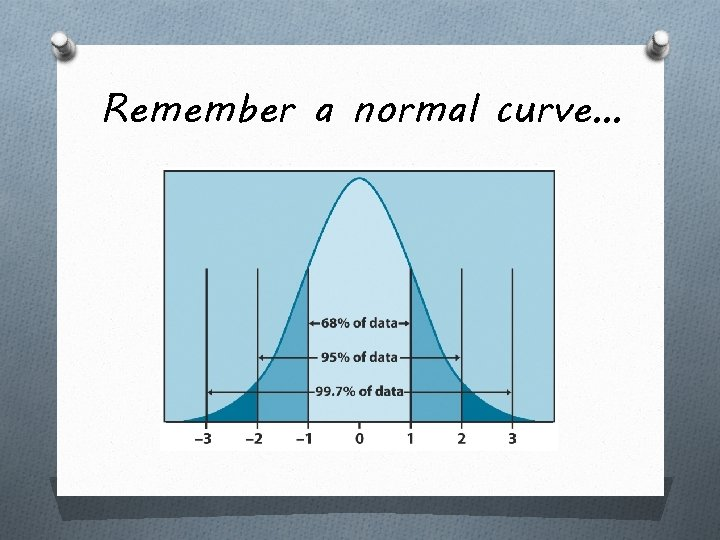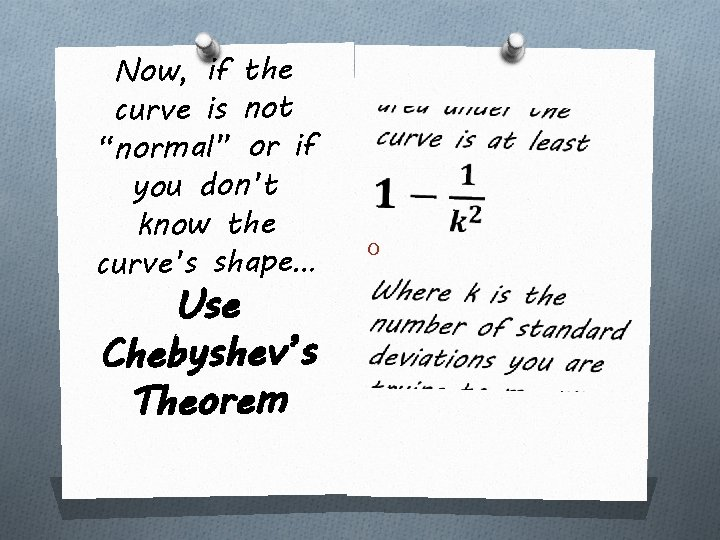# Calculating the Area Under the Curve Using the

• Slides: 7
Download presentationCalculating the Area Under the Curve Using the 68 -95 -99. 7 Rule or Chebyshev’s Theorem Chapter 5 LessonRemember a normal curve…From -1 to +1 = 68% of the data. So from -1 to 0 = 34% and From 0 to +1 = 34% of the data From -2 to +2 = 95% of the data. So from -2 to 0 = 47. 5% and From 0 to +2 = 47. 5% of the data. From -3 to +3 = 99. 7% of the data. So from -3 to 0 = 49. 85% and From 0 to +3 = 49. 85% of the data. So what is the measurement between each standard deviation?Answers… O -1 to 0 = 34% O 0 to +1 = 34% O -2 to -1 = 13. 5% O +1 to +2 = 13. 5% O -3 to -2 = 2. 35% O +2 to +3 = 2. 35% O On outer edges there is 0. 15% on each side (outlier data)And to label the curve… O The values along the bottom of the graph represent the 3 standard deviations to the right and left of the mean (the center number). O Simply add (to the right) or subtract (to the left) the standard deviation from the mean each time you move.Now, if the curve is not “normal” or if you don’t know the curve’s shape… Use Chebyshev’s Theorem OTomorrow. . O We will learn about z -scores which compare two numbers which are not on the same scale (like ACT and SAT scores). O How do you find the area under a curve using a z-score?